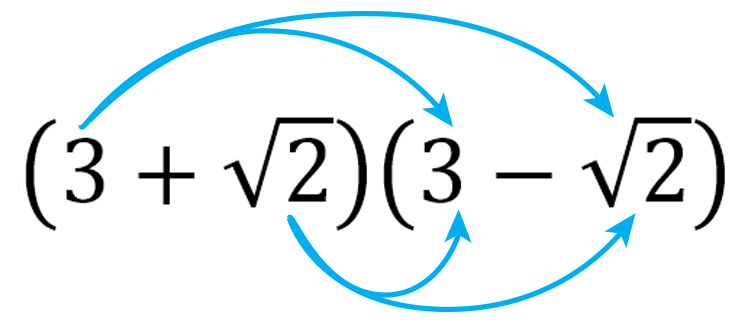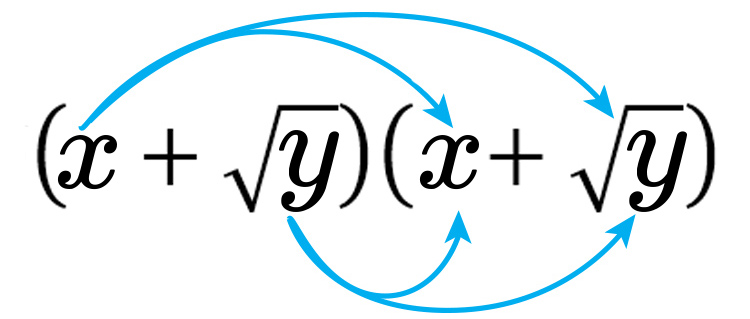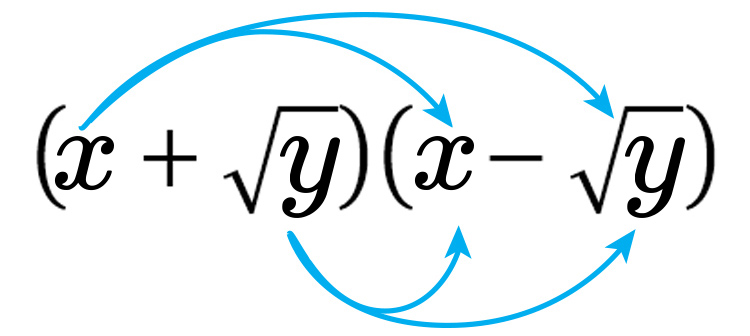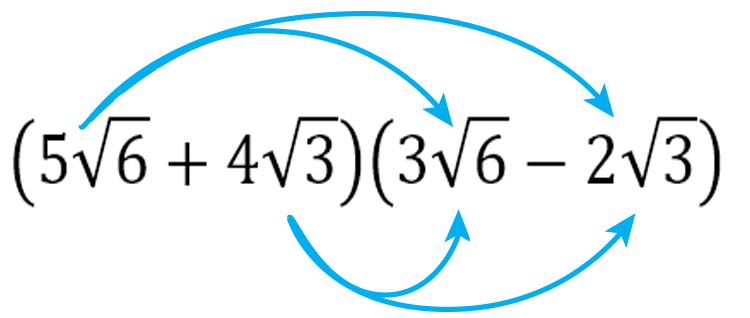# Difficult examples

1.  Simplify

4/sqrt8

The first thing you should attempt is to rationalise the denominator.

4/sqrt8=4/{sqrt8timessqrt8/sqrt8}=4/{{sqrt8timessqrt8}/sqrt8}=4/{{8}/sqrt8}

So we have rationalised the denominator

i.e.

4/sqrt8=4/(8/sqrt8)

But we can go further

4/{{8}/{sqrt8}}={4timessqrt8}/8=sqrt8/2

But now we can also simplify square roots

Simplify  sqrt8

Write down what we know

2=sqrt4,\ \ \ \  3=sqrt9,\ \ \ \  4=sqrt16

5=sqrt25,\ \  6=sqrt36,\ \  7=sqrt49

sqrt8=sqrt(4times2)

Try something we know using sqrt9

sqrt(9times4)=sqrt36=6

Is that the same as

sqrt9timessqrt4=3times2=6

Yes it is

Therefore sqrt(4times2)=sqrt4timessqrt2

sqrt4timessqrt2=2timessqrt2

To finish

sqrt8/2=(cancel2timessqrt2)/cancel(2)=sqrt2

Answer:  4/sqrt8=sqrt2

2.  Simplify sqrt(a^3)

Try something we know using sqrt9

We know sqrt(9^2) is the same as

sqrt9timessqrt9

i.e. both = 9

Therefore  sqrt(9^3)=sqrt9timessqrt9timessqrt9

Is the same as

=sqrt(9^2)timessqrt9=9sqrt9

Therefore  =sqrt(a^3)

Is the same as

=sqrt(a^2)timessqrta=asqrta

Answer:  sqrt(a^3)=asqrta

3.  Rationalise the denominator

2/(9sqrt5)

Rationalise the denominator = Turn the surd of the denominator into a fraction.

2/{9sqrt5}=2/{{9timessqrt5timessqrt5}/sqrt5}=2/{{9times5}/sqrt5}=2/{45/sqrt5}

We have rationalised the denominator but we can go further

2/{{45}/sqrt5}={2sqrt5}/45

Answer:  2/{9sqrt5}={2sqrt5}/45

4.  Simplify

(3+sqrt2)(3-sqrt2)

Multiply out=3times3+3times(-sqrt2)+3timessqrt2-sqrt2timessqrt2

=9-3sqrt2+3sqrt2 -sqrt(2times2)

=9-cancel(3sqrt2)+cancel(3sqrt2) -sqrt4

=9-2

= 7

5.  Simplify

(x+sqrty)^2

Multiply out=x^2+xsqrty+xsqrty+sqrtytimessqrty

=x^2+2xsqrty+sqrtytimessqrty

=x^2+2xsqrty+sqrt(y^2)

=x^2+2xsqrty+y

Answer:  =x^2+2xsqrty+y

6.  Simplify

(x+sqrty)(x-sqrty)

Multiply out=x^2-xsqrty+xsqrty-sqrtytimessqrty

=x^2-cancel(xsqrty)+cancel(xsqrty)-sqrt(y^2)

=x^2-y

Answer: =x^2-y

7.  Simplify

6sqrt2+sqrt18

First simplify

sqrt18

Write down what we know

2=sqrt4,\ \ \ \  3=sqrt9,\ \ \ \  4=sqrt16

5=sqrt25,\ \  6=sqrt36,\ \  7=sqrt49

The biggest square that divides into 18 is 9.

Therefore sqrt18 = sqrt(2times9)

Try something we know using  sqrt9

sqrt(4times9)=sqrt36=6

Is that the same as

sqrt4timessqrt9=3times2=6

Yes it is

Therefore sqrt(2times9)=sqrt2timessqrt9

sqrt2timessqrt9=3timessqrt2

So now getting back to the original question

6sqrt2+sqrt18

=6sqrt2+3sqrt2

Try something we know using sqrt9

6sqrt9+3sqrt9=6times3+3times3

=18+9=27

Is that the same as

(6+3)sqrt9=9sqrt9=9times3=27

Yes it is

Therefore  6sqrt2+3sqrt2

=(6+3)sqrt2

=9sqrt2

Answer:  6sqrt2+sqrt18=9sqrt2

8.  Simplify

8sqrt10times5sqrt15

8timessqrt10times5timessqrt15

8times5timessqrt10timessqrt15

40sqrt15timessqrt10

Try something we know using  sqrt9

sqrt9 timessqrt4=3times2=6

Is that the same as

sqrt(9 times4)=sqrt36=6

Yes it is

Therefore 40sqrt15timessqrt10

=40timessqrt(15times10)

=40sqrt150

So now simplify

sqrt150

Write down what we know

2=sqrt4,\ \ \ 3=sqrt9,\ \ \ \ \ \ 4=sqrt16

5=sqrt25,\ \  6=sqrt36,\ \ \ 7=sqrt49

8=sqrt64,\ \ 9=sqrt81,\ \ 10=sqrt100

The biggest square that divides into 150 = 25.

Therefore sqrt150=sqrt(3times25)=sqrt3timessqrt25

sqrt3timessqrt25=5sqrt3

Get back to

40sqrt150

This now equals

40times5sqrt3

Therefore 200sqrt3

Answer:  8sqrt10times5sqrt15=200sqrt3

9.  Simplify

(5sqrt6+4sqrt3)(3sqrt6-2sqrt3)

First multiply out the brackets5sqrt6times3sqrt6-2sqrt3times5sqrt6+4sqrt3times3sqrt6-2sqrt3times4sqrt3

Working on BIDMAS

## Stage 1

Work out  5sqrt6times3sqrt6

Try something we know using sqrt9

5sqrt9times3sqrt9=5times3times3times3

=15times9

Is that the same as

5times3timessqrt(9times9)=5times3timessqrt81

=15times9

Yes it is

Therefore 5timessqrt6times3timessqrt6

=5times3timessqrt6timessqrt6

=15sqrt36

=15times6

=90

## Stage 2

Work out  -2sqrt3times5sqrt6

Try something we know using  sqrt9

-2timessqrt9times5timessqrt4

=-2times3times5times2

=-6times10=-60

Is this the same as

-2times5timessqrt(9times4)

=-10timessqrt36

=-10times6=-60

Yes it is

Therefore -2timessqrt3times5timessqrt6

=-2times5timessqrt3timessqrt6

=-10timessqrt(3times6)

=-10timessqrt18

But we can simplify

sqrt18

Write down what we know

2=sqrt4,   3=sqrt9,   4=sqrt16

Therefore  sqrt18=sqrt(9times2)=sqrt9timessqrt2

sqrt18=3timessqrt2

Going back

Therfore -10timessqrt18

-10times3timessqrt2

-30sqrt2

## Stage 3

4sqrt3times3sqrt6

As stage 2

4timessqrt3times3timessqrt6

4times3timessqrt3times sqrt6

12timessqrt3timessqrt6

12timessqrt(3times6

12timessqrt18

We know from stage 2

sqrt18=3timessqrt2

Therefore  12timessqrt18=12times3timessqrt2=36sqrt2

## Stage 4

-2sqrt3times4sqrt3

As stage 2

-2timessqrt3times4timessqrt3

-2times4timessqrt3timessqrt3

-8timessqrt(3times3)

-8timessqrt9

-8times3

-24

Putting all the stages together:

90-30sqrt2+36sqrt2-24

90+6sqrt2-24

90-24+6sqrt2

66+6sqrt2

Answer:  (5sqrt6+4sqrt3)(3sqrt6-2sqrt3)

=66+6sqrt2